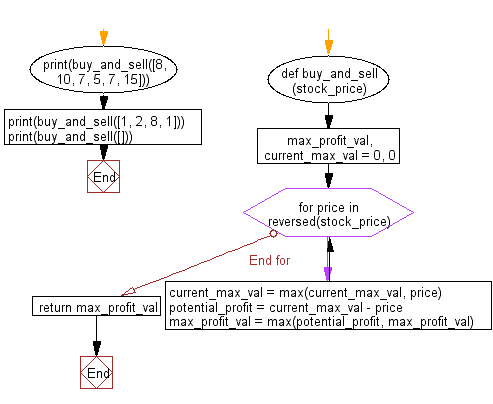﻿ Python: Calculate the maximum profit from selling and buying values of stock - w3resource# Python: Calculate the maximum profit from selling and buying values of stock

## Python Basic - 1: Exercise-74 with Solution

Write a Python program to calculate the maximum profit from selling and buying values of stock. An array of numbers represent the stock prices in chronological order.
For example, given [8, 10, 7, 5, 7, 15], the function will return 10, since the buying value of the stock is 5 dollars and sell value is 15 dollars.

Sample Solution:

Python Code:

``````def buy_and_sell(stock_price):
max_profit_val, current_max_val = 0, 0
for price in reversed(stock_price):
current_max_val = max(current_max_val, price)
potential_profit = current_max_val - price
max_profit_val = max(potential_profit, max_profit_val)

return max_profit_val

print(buy_and_sell([8, 10, 7, 5, 7, 15]))
``````

Sample Output:

```10
7
0
```

Flowchart:Python Code Editor:

Have another way to solve this solution? Contribute your code (and comments) through Disqus.

What is the difficulty level of this exercise?

Test your Programming skills with w3resource's quiz.

﻿

## Python: Tips of the Day

What is the difference between Python's list methods append and extend?

append: Appends object at the end.

```x = [1, 2, 3]
x.append([4, 5])
print (x)
```

Output:

```[1, 2, 3, [4, 5]]
```

extend: Extends list by appending elements from the iterable.

```x = [1, 2, 3]
x.extend([4, 5])
print (x)
```

Output:

```[1, 2, 3, 4, 5]
```

Ref: https://bit.ly/2AZ6ZFq